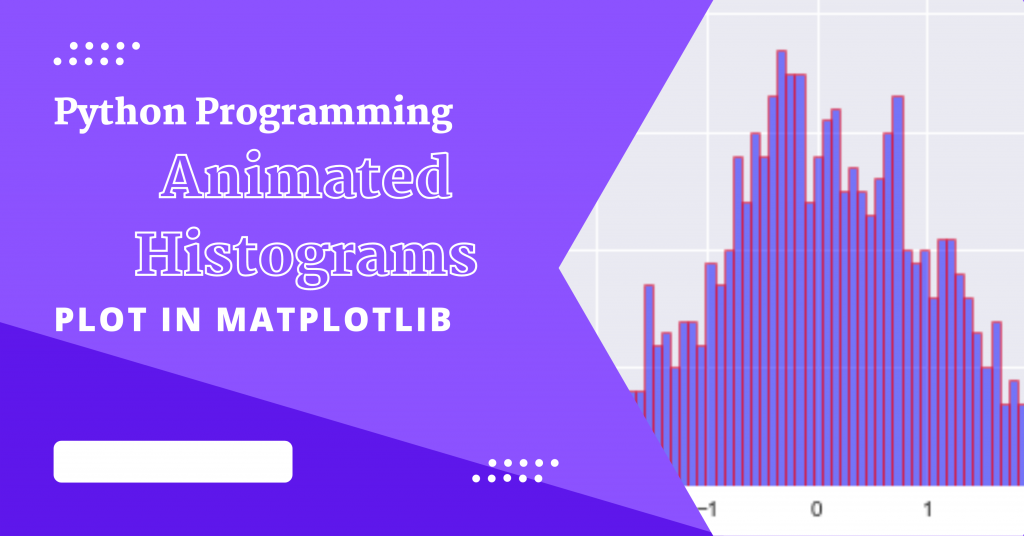# Animated Histograms in Python – A Step-By-Step ImplementationHey folks! Today, we are going to program in order to obtain animated histograms using the Python programming language.

Python and Matplotlib can be used to create static 2D plots. But Matplotlib has a secret power that can also be used to create dynamic auto-updating animated plots.

Let’s get started!

## 1. Importing Modules

We start off by importing all necessary modules/libraries which included `numpy` to create data, `matplotlib` in order to plot histograms and finally `matplotlib.animation` to plot animated plots.

We will also be importing the HTML function in order to convert the video to the HTML form.

```import numpy as np
import matplotlib.pyplot as plt
import matplotlib.animation as animation
from IPython.display import HTML
plt.style.use('seaborn')
```

## 2. Create a Dataset

In order to create data, we will be needing the numpy module by first fixing a random state in order to work with it. Next, we initialize the number of bins using the linespace function.

Next, we will create random 1000 data points using the linspace function. The final step is to convert the data points to histogram data points using the histogram function.

```np.random.seed(19680801)
HIST_BINS = np.linspace(-4, 4, 100)
data = np.random.randn(1000)
n, _ = np.histogram(data, HIST_BINS)
```

## 3. Animating the Histogram

In order to animate the histogram, we need an `animate` function, that will generate some random numbers and keep updating the heights of the bins.

```def prepare_animation(bar_container):

def animate(frame_number):
data = np.random.randn(1000)
n, _ = np.histogram(data, HIST_BINS)

for count, rect in zip(n, bar_container.patches):
rect.set_height(count)

return bar_container.patches

return animate
```

## 3. Display Animated Histograms

With the help of the `hist()` function, one can get an instance of `BarContainer`( Collection of Rectangle instances ).

Then we will be calling the `prepare_animation` , which has the `animate` function defined under it.

Finally, we will be converting the plot into HTML format, using the `to_html5_video` function.

```fig, ax = plt.subplots()
_, _, bar_container = ax.hist(data, HIST_BINS, lw=1,ec="red", fc="blue", alpha=0.5)
ax.set_ylim(top=55)
ani = animation.FuncAnimation(fig, prepare_animation(bar_container), 50,repeat=True, blit=True)
HTML(ani.to_html5_video())
```

## Complete Implementation to Display Animated Histograms in Python

```import numpy as np
import matplotlib.pyplot as plt
import matplotlib.animation as animation
from IPython.display import HTML
plt.style.use('seaborn')

np.random.seed(19680804)
HIST_BINS = np.linspace(-4, 4, 100)
data = np.random.randn(1000)
n, _ = np.histogram(data, HIST_BINS)

def prepare_animation(bar_container):

def animate(frame_number):
data = np.random.randn(1000)
n, _ = np.histogram(data, HIST_BINS)

for count, rect in zip(n, bar_container.patches):
rect.set_height(count)

return bar_container.patches

return animate

fig, ax = plt.subplots()
_, _, bar_container = ax.hist(data, HIST_BINS, lw=1,ec="blue", fc="yellow", alpha=0.5)
ax.set_ylim(top=100)
ani = animation.FuncAnimation(fig, prepare_animation(bar_container), 50,repeat=True, blit=True)
HTML(ani.to_html5_video())
```

## Conclusion

I hope you had fun watching the animated histograms! You can try this with different data, number of bins, and even change the speed of the histograms as well.

Happy Coding! 😊